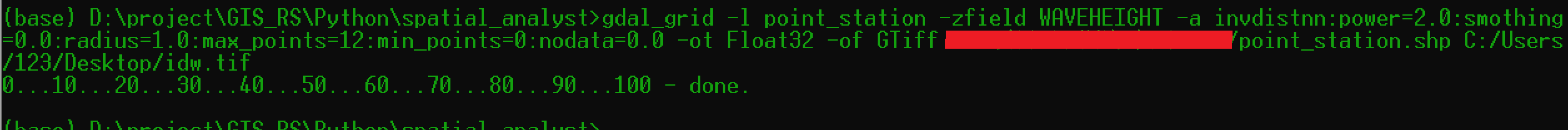• python空间
千次阅读
2018-04-26 09:45:34

# Python空间数据处理环境搭建

作者：阿振

邮箱：tanzhenyugis@163.com

修改时间：2018-04-26

## Conda的下载和安装

什么是Conda? 官方定义：Package, dependency and environment management for any language—Python, R, Ruby, Lua, Scala, Java, JavaScript, C/ C++, FORTRAN

Conda就是一个虚拟环境和包（库）依赖管理工具

对于Windows版本的，确定Python版本和系统类型直接下载安装包进行安装

对于Linux和macOS系统，在Terminal中运行bash脚本进行安装即可。

## Conda的使用

### 新建虚拟环境（Managing environments）

conda create -n osgeo

### 切换到新建的虚拟环境

source activate osgeo （Linux和macOS）

activate osgeo （Windows）

### 退出虚拟环境

source deactivate （Linux和macOS）

deactivate （Windows）

实用命令：

• 查看虚拟环境列表 conda env list 或者 conda info --envs
• 删除虚拟环境 conda remove --name <environment> --all
• 查看虚拟环境中的包列表 conda list
• 更新conda或者某个包 conda update conda 或者 conda update <package>
• 更新虚拟环境下的所有包 conda update --all
• 查看过时的包 conda search --outdated
• 搜索指定包 conda search <package>
• 删除某个包 conda remove <package>
• 添加channel到conda配置文件 conda config --add channels <channel> 或者 conda config --append channels <channel>

## 空间数据处理Python库的安装

### 常用的空间数据处理Python库

• GDAL 全能型的基础空间数据处理库
• fiona 基于GDAL的空间矢量数据处理库
• rasterio 基于GDAL的空间栅格处理库
• basemap 基于matplotlib的空间制图库
• GeoPandas 基于pandas的空间数据分析库
• RSGISlib 针对遥感数据及GIS分析的高级库

### 使用conda进行库的安装

打开命令行工具（Terminal），输入命令，进入虚拟环境

1. 安装GDAL库

conda install -c conda-forge gdal

2. 安装fiona库

conda install -c conda-forge fiona

3. 安装rasterio库

conda install -c conda-forge rasterio

### 使用pip进行库的安装

什么是pip呢？pip是Python默认和推荐实用的包管理工具，可以利用pip从PyPI网络仓库自动下载Python包进行安装和管理。

对于Windows下的二进制库的预编译包，提供给大家一个网站：Unofficial Windows Binaries for Python Extension Packages

使用pip安装的时候，如果该Python包底层依赖一些C++库，则需要手动进行编译，或者安装指定平台下预编译好的库。

1. 安装GDAL库

pip install GDAL‑2.2.4‑cp37‑cp37m‑win_amd64.whl

2. 安装fiona库

pip install Fiona‑1.7.11.post1‑cp37‑cp37m‑win_amd64.whl

3. 安装rasterio库

pip install rasterio‑1.0a12‑cp37‑cp37m‑win_amd64.whl

4. 安装Jupyter

pip install jupyter

使用Jupyter Notebook进行编程

jupyter notebook

更多相关内容
• 全书共分为10 章，分别介绍了Python与地理空间分析、地理空间数据、地理空间技术、Python的地理空间分析 工具、Python与地理信息系统、Python与遥感、Python与高程数据、Python与地理空间高级建模、 实时数据、综合...
• #三维点插值#在三维空间中，利用实际点的值推算出网格点的值import numpy as np point_grid =np.array([[0.0,0.0,0.0],[0.4,0.4,0.4],[0.8,0.8,0.8],[1.0,1.0,1.0]])#网格点坐标 def func(x, y, z): return x*(1...
#三维点插值#在三维空间中，利用实际点的值推算出网格点的值import numpy as np

point_grid =np.array([[0.0,0.0,0.0],[0.4,0.4,0.4],[0.8,0.8,0.8],[1.0,1.0,1.0]])#网格点坐标

def func(x, y, z):
return x*(1-x)*np.cos(4*np.pi*x) * (np.sin(4*np.pi*y**2)**2)*z

points = np.random.rand(10, 3)#实际点坐标
values = func(points[:,0], points[:,1],points[:,2])#实际点的值

from scipy.interpolate import griddata
grid_z0 = griddata(points, values, point_grid, method='nearest')#插值计算，计算出网格点的值

展开全文•    近几年，基于位置服务的应用层出不穷，如GPS车辆导航、打车、外卖、运动等，产生了大量的具有时空信息的轨迹数据，对...本课程讲述了Python对时空数据的处理，以及机器学习方法在空间数据处理上的应用。
• 随机子空间法的python实现，用于振动信号模态识别
• Python地理空间分析指南第2版，完整版，是由epub格式转换而来，值得学习！
• Python地理空间分析指南 第2版 pdf文档
• 基于Python实现全局和局部双变量Moran指数计算，输入参数可直接是shapefile文件。
• usr/bin/env python # -*- coding: utf-8 -*- """ @Author : zhaoguanhua @Email : @Time : 2020/3/6 14:11 @File : Wave_Interpolation.py @Software: PyCharm """ """ 基于站点数据，使用IDW插值生成海浪面数据，...

使用idw插值法对站点观测数据进行面插值。
使用方法
1、gdal_grid.exe2、gdal.grid()

opts = gdal.GridOptions(algorithm="invdistnn:power=2.0:smothing=0.0:radius=1.0:max_points=12:min_points=0:nodata=0.0",
format="GTiff",outputType=gdal
展开全文gdal
• 在编写Python中的自定义函数或算法时，减低时间复杂度和空间复杂度，会大大提升Python自定义函数或算法的整体性能。 时间复杂度 时间复杂度是用来估计自定义函数或算法运行时间的一个式子（单位）,时间复杂度常用“O...

# 简介

在编写Python中的自定义函数或算法时，减低时间复杂度空间复杂度，会大大提升Python自定义函数或算法的整体性能，提升性能的几个书写好习惯

• 尽量不要写循环，最好是一次就输出结果
• 如果写循环，尽量写一层循环就能有结果
• 避免嵌套

# 时间复杂度

时间复杂度是用来估计自定义函数或算法运行时间的一个式子（单位）,时间复杂度常用“O”表述，而且时间复杂度可被称为是渐近的，它考察当输入值大小趋近 ∞ \infin 时的情况

时间复杂度为 O(1)的例子

print('Hello!')
print(1)


时间复杂度为 O(n)的例子

# 其中range(n) 换成range(length(n))或者直接换成n，其时间复杂度都为 O(n)
for i in range(n):
print('Hello!')


时间复杂度为O(n^2)的例子

# 其中range(n) 换成range(length(n))或者直接换成n，其时间复杂度都为 O(n^2)
for i in range(n):
for j in range(n):
print('Hello!')

for i in range(n):
print('Hello!')
for j in range(n):
print('Hello!')

for i in range(n):
for j in range(i):
print('Hello!')


时间复杂度为O(n^3)的例子

# 其中range(n) 换成range(length(n))或者直接换成n，其时间复杂度都为 O(n^3)
for i in range(n):
for j in range(n):
for k in range(n):
print('Hello!')


由上述时间复杂度O(n)的变化可以得出这样的结论：O(n)中n的几次方取决于自定义函数或算法中存在几个循环

时间复杂度为O(n^n)的例子

# 其中range(n) 换成range(length(n))或者直接换成n，其时间复杂度都为 O(n^n)
for i in range(n):
for j in range(n):
for k in range(n):
for l in range(n):
.
.
.
for o in range(n):
print('Hello!')


时间复杂度为O( l o g 2 n log_2n )的例子

n = 128
while n > 1:
print(n)
n = n // 2 # //表示整除法


计算上述问题的时间复杂度步骤：

1. 2 7 = 128 2^7 = 128
2. l o g 2 128 = 7 log_2128 = 7
3. 循环减半的时间复杂度为O( l o g 2 128 log_2128 )，即O( l o g n 128 log_n128 ) = O(7)

复杂度为O( l o g 2 n log_2n )

n = n
while n > 1:
print(n)
n = n // 2 # //表示整除法


常见的时间复杂度高低排序：O(1)<O( l o g n log_n )<O(n)<O( n l o g n nlog_n )<O( n 2 n^2 )<O( n 2 l o g n n^2log_n )<O( n 3 n^3 )

# 空间复杂度

空间复杂度：用来评估自定义函数或算法内存占用大小

空间复杂度为 1的例子

p = 'Python'
l = 'like'
m = 'me'


空间复杂度为 n

p = [1,2,3,....,n]


空间复杂度为 n*n

p_n = [[1,2,3,....,n],[1,2,3,....,n],.....,[1,2,3,....,n]]


空间复杂度为 n * n * n

p_n = [[[1,2,3,....,n],[1,2,3,....,n],.....,[1,2,3,....,n]],
[[1,2,3,....,n],[1,2,3,....,n],.....,[1,2,3,....,n]],
....,
[[1,2,3,....,n],[1,2,3,....,n],.....,[1,2,3,....,n]]


空间复杂度却决于：

• 变量的长度
• 变量的个数
• 变量的复杂程度

# 示例展示

自定义方差（variance）函数简化，以及计算其对应的时间复杂度和空间复杂度

def variance (*args): # *args不限定输入的可变量，这里输入的数量为n
total = 0 #时间复杂度为 1；空间复杂度为 1
average = float(sum(args))/len(args)
# 时间复杂度为 n+1 其中sum（args）为n，len(args)为 1
# 空间复杂度为 1
for val in args:
total +=(val-average)**2
# 时间复杂度为 n，即进行了n次循环
# 空间复杂度为 n，即存储了n次total


时间复杂度：1+n+1+n+1 = 2n+1
空间复杂度：1+1+n+1 = n+3

展开全文• python处理数据 分析 基于Python的arcgis空间数据处理与分析
• 空间向量夹角1、原理2、代码及详解3、结果 4、 ★佐佑思维公众号提供更多学习学术服务★ 二维码如下： 1、原理 cos⁡<a⃗,b⃗>=a⃗⋅b⃗∣a⃗∣⋅∣b⃗∣=a1b1+a2b2+a3b3a12+a22+a32⋅b12+b22+b32 \cos <\...numpy
• 一种能有条不紊 、完备地保存遥感影像的属性和空间信息数据 , 同时使查询和提取相关数据也很方便容易的数据格式格式。HDF格式是一种较为常见的遥感数据格式（例如MODIS、3B43降水数据），但GDAL暂时未能给HDF格式...pyhdf gdal hdf格式
• python颜色空间转换。imgvision中cvtcolor()的用法opencv 计算机视觉
• 全书共分为10章，分别介绍了Python与地理空间分析、地理空间数据、地理空间技术、Python的地理空间分析工具、Python与地理信息系统、Python与遥感、Python与高程数据、Python与地理空间高级建模、实时数据、综合应用...
• 该函数是SpatialReference类的一个构造函数，它创建了一个SpatialReference类的对象，用以存储空间参考信息。 （2）object.ImportFromEPSG(4326) 该函数是SpatialReference类的一个成员函数，它从EPSG中导入一个空间...
• #coding:UTF-8 """ Python implementation of Haversine formula Copyright (C) <2009> Bartek Górny This program is free software: you can redistribute it and/or modify it under the terms of the GNU ...
• 全书共分为10章，分别介绍了Python与地理空间分析、地理空间数据、地理空间技术、Python的地理空间分析工具、Python与地理信息系统、Python与遥感、Python与高程数据、Python与地理空间高级建模、实时数据、综合应用...
• GDAL是空间数据处理的开源包，支持多种数据格式的读写。遥感图像是一种带大地坐标的栅格数据，遥感图像的栅格模型包含两部分的内容：栅格矩阵：由正方形或者矩形栅格点组成，每个栅格点所对应的数值为该点的像元值，...gdal 遥感影像处理
• 全书共分为10章，分别介绍了Python与地理空间分析、地理空间数据、地理空间技术、Python的地理空间分析工具、Python与地理信息系统、Python与遥感、Python与高程数据、Python与地理空间高级建模、实时数据、综合应用...
• 大O表示法 O 名称 举例 1 常量时间 一次赋值 logn 对数时间 折半查找 n 线性时间 线性查找 nlogn 对数线性时间 快速排序 n**2 平方 两重循环 n**3 ... 旅行商问...
• 基于网络的方法：这类方法的原理就是将数据空间划分为网格单元，将数据对象集映射到网格单元中，并计算每个单元的密度。根据预设的 密度阈值 判断每个网格单元是否为 高密度单元，由邻近的稠密单元组形成 “类”（簇...算法 聚类
• 前面两篇推文我们分别介绍了使用Python和R进行IDW(反距离加权法) 插值的计算及结果的可视化过程，详细内容可见如下：本期推文，我们将介绍如何使用Python进行克里金(Kriging)插值计算及插值结果的可视化绘制。...
• print detail_cont.find("table",id="locationdetails").textpython...